# How Autoencoders works ?

Autoencoders are the models in a dataset that find low-dimensional representations by exploiting the extreme non-linearity of neural networks. An autoencoder is made up of two parts:

Encoder – This transforms the input (high-dimensional into a code that is crisp and short.
Decoder – This transforms the shortcode into a high-dimensional input.

Assume that from a data-generating process,` pdata(x)`, if X is a set of samples drawn. Suppose xi >>n; however, do not keep any restrictions on the support structure. An example of this is, for RGB images, xi >> n×m×3.

Here is a simple illustration of a generic autoencoder: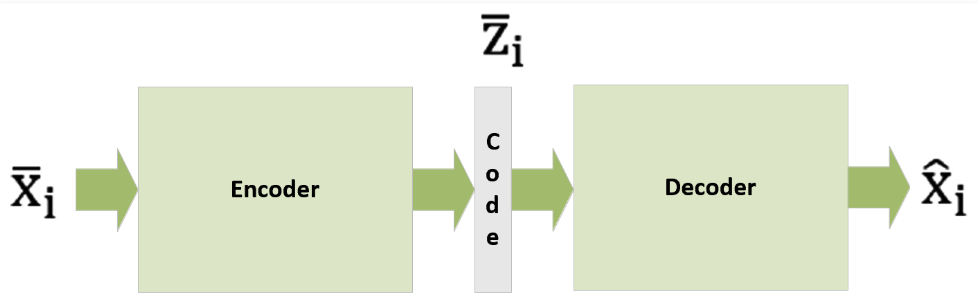For a p-dimensional vector code, a parameterized function, e(•) is the definition of the encoder: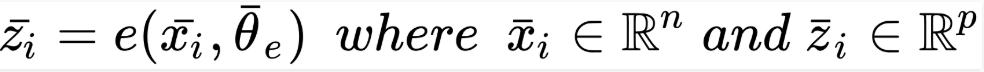In an analogous way, the decoder is another parameterized function, d(•):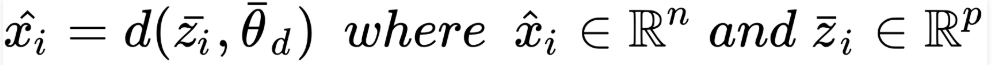Thus, when given an input sample, xi, a full autoencoder, an amalgamated function, will provide the best alternative as output: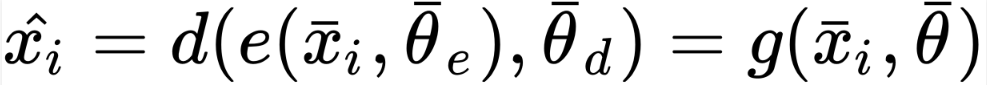An autoencoder is trained using the back-propagation algorithm frequently based on the mean square error cost function, the reason being an autoencoder is generally applied through neural networks.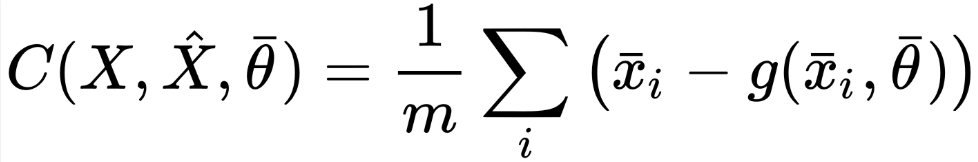On the other hand, if you consider the process of data generation, you can look at the parameterized conditional distribution, q(•) to reiterate the objective: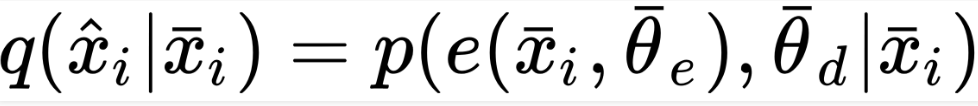This results in the cost function to develop into the Kullback-Leibler divergence between pdata(•) and q(•):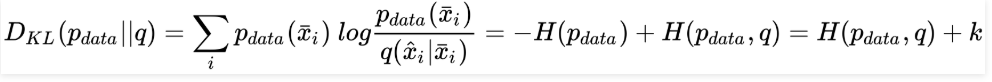Using the optimization process, pdata can be excluded, as its entropy is constant. Now, the minimization of the cross-entropy between pdata and q and divergence is equal. The Kullback-Leibler cost function and the mean square error are equal. If you assume that pdata and q are Gaussian, you can interchange both methods of approach.

In some cases, you can implement a Bernoulli distribution for either pdata and q. But, this is only possible when you normalize the range of data to (0, 1). This is not entirely correct on a formal note though as a Bernoulli distribution is binary and `xi ? {0, 1}d`. The use of sigmoid output units will also result in an effective optimization for continuous samples, `xi? (0, 1)d`. Now, the cost function will look as follows: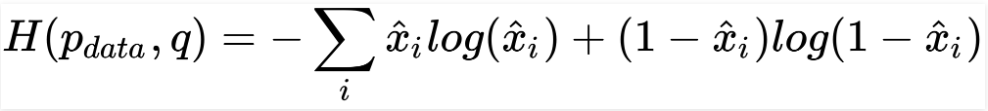### Implementing a deep convolutional autoencoder –

Now let’s look at an example of a TensorFlow-based deep convolutional autoencoder. We’ll use the Olivetti faces dataset as it small in size, fits the purposes, and contains many expressions.

Step #1: Load the 400 64 × 64 grayscale image samples to prepare the set for training:

 `from` `sklearn.datasets ``import` `fetch_olivetti_faces ` ` `  `faces ``=` `fetch_olivetti_faces(shuffle``=``True``, random_state``=``1000``) ` `X_train ``=` `faces[``'images'``] `

Step #2: Now, to increase the speed of our computation, we will resize them to 32 × 32. This will also help avoid any memory issues. We may lose a minor visual precision. Note that you can skip this if you have high computational resources.

Step #3: Let’s define the main constants.

```-> number of epochs (nb_epochs)
-> batch_size
-> code_length
-> graph```

 `import` `tensorflow as tf ` ` `  `nb_epochs ``=` `600` `batch_size ``=` `50` `code_length ``=` `256`  `width ``=` `32` `height ``=` `32` ` `  `graph ``=` `tf.Graph() `

Step #4: Using 50 samples per batch, we will now train the model for 600 epochs. With images size of 64 × 64 = 4, 096, we’ll get the compression ratio of 4, 096/256 = 16 times. You can always try different configurations for maximizing convergence speed and the ultimate accuracy.

Step #5: Model the encoder with these layers.

-> 2D convolution with 16 (3 × 3) filters, (2 × 2) strides, ReLU activation, and the same padding.
-> 2D convolution with 32 (3 × 3) filters, (1 × 1) strides, ReLU activation, and the same padding.
-> 2D convolution with 64 (3 × 3) filters, (1 × 1) strides, ReLU activation, and the same padding.
-> 2D convolution with 128 (3 × 3) filters, (1 × 1) strides, ReLU activation, and the same padding.

Step #6: The decoder achieves deconvolutions (a sequence of transpose convolutions).

-> 2D transpose convolution with 128 (3 × 3) filters, (2 × 2) strides, ReLU activation, and the same padding.
-> 2D transpose convolution with 64 (3 × 3) filters, (1 × 1) strides, ReLU activation, and the same padding.
-> 2D transpose convolution with 32 (3 × 3) filters, (1 × 1) strides, ReLU activation, and the same padding.
-> 2D transpose convolution with 1 (3 × 3) filter, (1 × 1) strides, Sigmoid activation, and the same padding.

The loss function is based on the L2 norm of the difference between the reconstructions and the original images. Here, Adam is the optimizer with a learning rate of α =0.001. Now, let’s look at the encoder part of the TensorFlow DAG:

 `import` `tensorflow as tf ` ` `  `with graph.as_default(): ` `    ``input_images_xl ``=` `tf.placeholder(tf.float32,  ` `                                     ``shape``=``(``None``, X_train.shape[``1``], ` `                                     ``X_train.shape[``2``], ``1``)) ` ` `  `    ``input_images ``=` `tf.image.resize_images(input_images_xl, ` `                                          ``(width, height), ` `                    ``method``=``tf.image.ResizeMethod.BICUBIC) ` ` `  `    ``# Encoder ` `    ``conv_0 ``=` `tf.layers.conv2d(inputs``=``input_images, ` `                              ``filters``=``16``, ` `                              ``kernel_size``=``(``3``, ``3``), ` `                              ``strides``=``(``2``, ``2``), ` `                              ``activation``=``tf.nn.relu, ` `                              ``padding``=``'same'``) ` ` `  `    ``conv_1 ``=` `tf.layers.conv2d(inputs``=``conv_0, ` `                              ``filters``=``32``, ` `                              ``kernel_size``=``(``3``, ``3``), ` `                              ``activation``=``tf.nn.relu, ` `                              ``padding``=``'same'``) ` ` `  `    ``conv_2 ``=` `tf.layers.conv2d(inputs``=``conv_1, ` `                              ``filters``=``64``, ` `                              ``kernel_size``=``(``3``, ``3``), ` `                              ``activation``=``tf.nn.relu, ` `                              ``padding``=``'same'``) ` ` `  `    ``conv_3 ``=` `tf.layers.conv2d(inputs``=``conv_2, ` `                              ``filters``=``128``, ` `                              ``kernel_size``=``(``3``, ``3``), ` `                              ``activation``=``tf.nn.relu, ` `                              ``padding``=``'same'``) `

The following is the coding part of the DAG:

 `import` `tensorflow as tf ` ` `  `with graph.as_default():  ` `   `  `    ``# Code layer ` `    ``code_input ``=` `tf.layers.flatten(inputs``=``conv_3) ` ` `  `    ``code_layer ``=` `tf.layers.dense(inputs``=``code_input, ` `                                 ``units``=``code_length, ` `                                 ``activation``=``tf.nn.sigmoid) ` ` `  `    ``code_mean ``=` `tf.reduce_mean(code_layer, axis``=``1``) `

Now, let’s look at the DAG decoder:

 `import` `tensorflow as tf ` ` `  `with graph.as_default():  ` ` `  `    ``# Decoder ` `    ``decoder_input ``=` `tf.reshape(code_layer, ` `                      ``(``-``1``, ``int``(width ``/` `2``), ` `                       ``int``(height ``/` `2``), ``1``)) ` ` `  `    ``convt_0 ``=` `tf.layers.conv2d_transpose(inputs``=``decoder_input, ` `                                         ``filters``=``128``, ` `                                         ``kernel_size``=``(``3``, ``3``), ` `                                         ``strides``=``(``2``, ``2``), ` `                                         ``activation``=``tf.nn.relu, ` `                                         ``padding``=``'same'``) ` ` `  `    ``convt_1 ``=` `tf.layers.conv2d_transpose(inputs``=``convt_0, ` `                                         ``filters``=``64``, ` `                                         ``kernel_size``=``(``3``, ``3``), ` `                                         ``activation``=``tf.nn.relu, ` `                                         ``padding``=``'same'``) ` ` `  `    ``convt_2 ``=` `tf.layers.conv2d_transpose(inputs``=``convt_1, ` `                                         ``filters``=``32``, ` `                                         ``kernel_size``=``(``3``, ``3``), ` `                                         ``activation``=``tf.nn.relu, ` `                                         ``padding``=``'same'``) ` ` `  `    ``convt_3 ``=` `tf.layers.conv2d_transpose(inputs``=``convt_2, ` `                                         ``filters``=``1``, ` `                                         ``kernel_size``=``(``3``, ``3``), ` `                                         ``activation``=``tf.sigmoid, ` `                                         ``padding``=``'same'``) ` ` `  `    ``output_images ``=` `tf.image.resize_images(convt_3, (X_train.shape[``1``], ` `                                                    ``X_train.shape[``2``]),  ` `                                  ``method``=``tf.image.ResizeMethod.BICUBIC) `

Step #7: Here’s how you define the loss function and the Adam optimizer –

 `import` `tensorflow as tf ` ` `  `with graph.as_default(): ` `    ``# Loss ` `    ``loss ``=` `tf.nn.l2_loss(convt_3 ``-` `input_images) ` ` `  `    ``# Training step ` `    ``training_step ``=` `tf.train.AdamOptimizer(``0.001``).minimize(loss) `

Step #8: Now that we have defined the full DAG, we can start the session and initialize all variables.

 `import` `tensorflow as tf ` ` `  `session ``=` `tf.InteractiveSession(graph``=``graph) ` `tf.global_variables_initializer().run() `

Step #9: We can start the training process after the initialization of TensorFlow:

 `import` `numpy as np ` ` `  `for` `e ``in` `range``(nb_epochs): ` `    ``np.random.shuffle(X_train) ` ` `  `    ``total_loss ``=` `0.0` `    ``code_means ``=` `[] ` ` `  `    ``for` `i ``in` `range``(``0``, X_train.shape[``0``] ``-` `batch_size, batch_size): ` `        ``X ``=` `np.expand_dims(X_train[i:i ``+` `batch_size, :, :], ` `                                ``axis``=``3``).astype(np.float32) ` ` `  `        ``_, n_loss, c_mean ``=` `session.run([training_step, loss, code_mean], ` `                                        ``feed_dict``=``{input_images_xl: X}) ` ` `  `        ``total_loss ``+``=` `n_loss ` `        ``code_means.append(c_mean) ` ` `  `    ``print``(``'Epoch {}) Average loss per sample: {} (Code mean: {})'``. ` `          ``format``(e ``+` `1``, total_loss ``/` `float``(X_train.shape[``0``]), ` `          ``np.mean(code_means))) `

Output:

```Epoch 1) Average loss per sample: 11.933397521972656 (Code mean: 0.5420681238174438)
Epoch 2) Average loss per sample: 10.294102325439454 (Code mean: 0.4132006764411926)
Epoch 3) Average loss per sample: 9.917563934326171 (Code mean: 0.38105469942092896)
...
Epoch 600) Average loss per sample: 0.4635812330245972 (Code mean: 0.42368677258491516)```

When the training process culminates, 0.46 (considering 32 × 32 images) is the average loss per sample and 0.42 is the mean of the codes. This proves that the encoding is relatively dense bringing the average to 0.5. Our focus is to look at sparsity during the comparison of the result.

Some sample images have resulted in the following output of the autoencoder: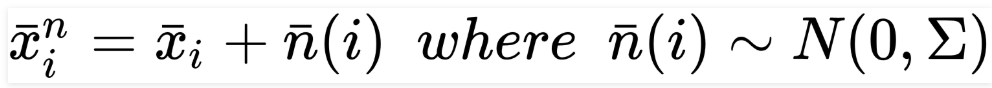When the images are upsized to 64 × 64, the quality of the reconstruction is affected partially. However, we can reduce the compression ratio and increase the code length to get better results.

How to denoise autoencoders ?
An application of autoencoders can be found helpful when it depends on the process of transformation from input to output. It is not necessarily related to autoencoder’s ability to find lower-dimensional representations.

Let’s take a look at an example where we assume X as a zero-centered dataset and a noisy version, the sample of which will have a structure as follows: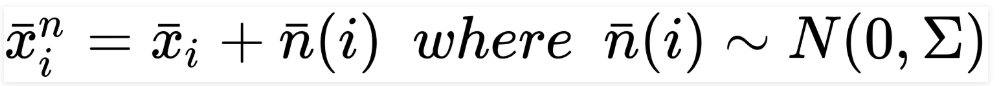Here, the autoencoder’s focus is to remove the noisy term and bring back the original sample, xi. If we look at this from a mathematical perspective, standard and denoising autoencoders are one and the same but we need to look at the capacity needs for considering these models. As they have to recover original samples, given a corrupted input (whose features occupy a much larger sample space), the amount and dimension of layers might be larger than for a standard autoencoder.

Of course, considering the complexity, it’s impossible to have clear insight without a few tests; therefore, I strongly suggest starting with smaller models and increasing the capacity until the optimal cost function reaches a suitable value. You can use various strategies to add noise such as, corrupting the samples contained in each batch, using a noise layer as input 1 for the encoder, or using a dropout layer as input 1 for the encoder.

One of the most collective choices is to assume that the noise is Gaussian. If so, we can create homoscedastic and heteroscedastic noise. The first case has a variance that is kept constant for all components (that is, n(i) ? N(0, ?2I)), while the second case has components that have their own variance. Looking at the nature of the issue, we can choose another apt solution. But, it is preferable to implement heteroscedastic noise to improve the sturdiness of the entire system.

### How to add noise to autoencoders –

We will modify our deep convolutional autoencoder so that it can manage noisy input samples. Since the DAG is almost equal, we need to include images that original and noisy.

 `import` `tensorflow as tf ` ` `  `with graph.as_default(): ` `    ``input_images_xl ``=` `tf.placeholder(tf.float32,  ` `                         ``shape``=``(``None``, X_train.shape[``1``], ` `                         ``X_train.shape[``2``], ``1``)) ` `    ``input_noisy_images_xl ``=` `tf.placeholder(tf.float32,  ` `                             ``shape``=``(``None``, X_train.shape[``1``], ` `                             ``X_train.shape[``2``], ``1``)) ` ` `  `    ``input_images ``=` `tf.image.resize_images(input_images_xl,  ` `                                          ``(width, height),  ` `                     ``method``=``tf.image.ResizeMethod.BICUBIC) ` ` `  `    ``input_noisy_images ``=` `tf.image.resize_images(input_noisy_images_xl, ` `                                                      ``(width, height),  ` `                                 ``method``=``tf.image.ResizeMethod.BICUBIC) ` ` `  `    ``# Encoder ` `    ``conv_0 ``=` `tf.layers.conv2d(inputs``=``input_noisy_images, ` `                              ``filters``=``16``, ` `                              ``kernel_size``=``(``3``, ``3``), ` `                              ``strides``=``(``2``, ``2``), ` `                              ``activation``=``tf.nn.relu, ` `                              ``padding``=``'same'``) `

Considering the new images, we can calculate the loss function:

 `# Loss ` `loss ``=` `tf.nn.l2_loss(convt_3 ``-` `input_images) ` ` `  `# Training step ` `training_step ``=` `tf.train.AdamOptimizer(``0.001``).minimize(loss) `

Once the standard initialization of the variables is complete, we can start the training process considering an additive noise, ni ? N(0, 0.45) (that is, ? ? 0.2):

 `import` `numpy as np ` ` `  `for` `e ``in` `range``(nb_epochs): ` `    ``np.random.shuffle(X_train) ` ` `  `    ``total_loss ``=` `0.0` `    ``code_means ``=` `[] ` ` `  `    ``for` `i ``in` `range``(``0``, X_train.shape[``0``] ``-` `batch_size, batch_size): ` `        ``X ``=` `np.expand_dims(X_train[i:i ``+` `batch_size, :, :], ` `                                 ``axis``=``3``).astype(np.float32) ` ` `  `        ``Xn ``=` `np.clip(X ``+` `np.random.normal(``0.0``, ``0.2``,  ` `                     ``size``=``(batch_size, X_train.shape[``1``], ` `                     ``X_train.shape[``2``], ``1``)), ``0.0``, ``1.0``) ` ` `  `        ``_, n_loss, c_mean ``=` `session.run([training_step, loss, code_mean], ` `                                       ``feed_dict``=``{ input_images_xl: X, ` `                                             ``input_noisy_images_xl: Xn }) ` ` `  `        ``total_loss ``+``=` `n_loss ` `        ``code_means.append(c_mean) ` ` `  `    ``print``(``'Epoch {}) Average loss per sample: {} (Code mean: {})'``. ` `                ``format``(e ``+` `1``, total_loss ``/` `float``(X_train.shape[``0``]), ` `                                             ``np.mean(code_means))) `

Now that we have trained the model, we use some noisy samples to test it. Here is the output of this action –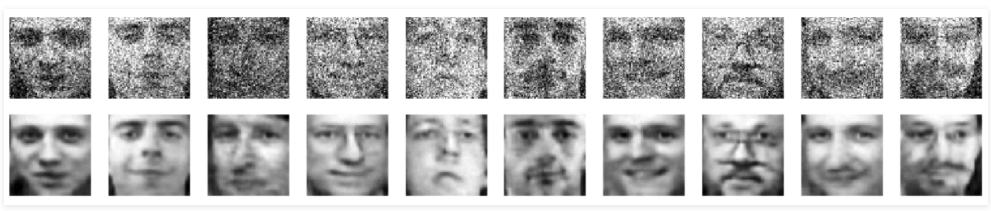We have successfully taught the autoencoder to denoise the input images, no matter the quality of the input images. You can go ahead and try different datasets to achieve maximum noise variance.

Reference:
Hands-On Unsupervised Learning with Python.

Attention geek! Strengthen your foundations with the Python Programming Foundation Course and learn the basics.

To begin with, your interview preparations Enhance your Data Structures concepts with the Python DS Course.

My Personal Notes arrow_drop_upWe are one of the leading global learning solution providers for IT professionals, having published over 6000 books and videos till date Our books, courses and videos are created with the aim to make IT simpler, addressing day-to-day issues that developers may face in their job

If you like GeeksforGeeks and would like to contribute, you can also write an article using contribute.geeksforgeeks.org or mail your article to contribute@geeksforgeeks.org. See your article appearing on the GeeksforGeeks main page and help other Geeks.

Please Improve this article if you find anything incorrect by clicking on the "Improve Article" button below.

Article Tags :
Practice Tags :

Be the First to upvote.

Please write to us at contribute@geeksforgeeks.org to report any issue with the above content.